# Programmable-Gain Transimpedance Amplifiers Maximize Dynamic Range in Spectroscopy Systems

### Introduction

Precision instrumentation systems that measure physical properties using a photodiode or other current-output sensor often include a transimpedance amplifier (TIA) and a programmable-gain stage to maximize dynamic range. This article uses a real-world example to show the benefits and challenges of implementing a single-stage programmable-gain TIA to minimize noise while maintaining high bandwidth and high accuracy.

Transimpedance amplifiers are essential building blocks in any system that measures light. Many chemical analysis instruments, such as ultraviolet-visible (UV-VIS) or Fourier transform-infrared (FT-IR) spectroscopes, rely on photodiodes to accurately identify chemical compounds. These systems must measure a wide range of light intensity. For example, a UV-VIS spectroscope can measure opaque samples, such as used motor oil, or transparent substances, such as ethanol. In addition, some substances have strong absorption bands at certain wavelengths, while remaining almost transparent at other wavelengths. Instrument designers often add several programmable gains to the signal path to increase the dynamic range.

### Photodiodes and Photodiode Amplifiers

Before discussing photodiode amplifiers, let’s start with a quick refresher on photodiodes, which are devices that generate voltage or current when light hits their PN junction. Figure 1 shows the equivalent circuit. For a typical device used in spectroscopy, the model includes a light-dependent current source in parallel with a large shunt resistor and a shunt capacitor that can range from less than 50 pF for small devices to more than 5000 pF for very large devices.

Figure 2 shows the transfer function for a typical photodiode. The curve looks very similar to that of a normal diode, but the entire curve moves up and down as the photodiode is exposed to light. Figure 2b shows a close-up of the transfer function around the origin, where no light is present. The output of the photodiode is nonzero as long as the bias voltage is nonzero. This dark current is typically specified with a 10-mV reverse bias. Although operating the photodiode with a large reverse bias (photoconductive mode) results in a faster response, operating with zero bias (photovoltaic mode) eliminates the dark current. In practice, the dark current does not disappear completely, even in photovoltaic mode, as the amplifier’s input offset voltage will result in a small error across the photodiode’s terminals.

When operating a photodiode in photovoltaic mode, a transimpedance amplifier (TIA) keeps the bias voltage near 0 V while converting the photodiode current to a voltage. Figure 3 shows the most basic form of a TIA.

### DC Error Sources

With an ideal op amp, the inverting input of the amplifier will be at virtual ground, and all of the photodiode current will flow through the feedback resistor, Rf. With one end of Rf at virtual ground, the output voltage is simply Rf × Id. In order for this approximation to hold true, the op amp’s input bias current and input offset voltage must be small. In addition, a small input offset voltage will minimize the photodiode’s dark current. A good amplifier choice is the AD8615, which specifies 1-pA maximum leakage and 100-μV maximum offset at room temperature. In this example, we choose Rf = 1 MΩ to provide the desired output level with the maximum light input.

Unfortunately, designing a photodiode amplifier is not as simple as selecting an op amp for the circuit shown in Figure 3. If we simply connect Rf = 1 MΩ across the feedback path of the op amp, the photodiode’s shunt capacitance will cause the op amp to oscillate. To illustrate this, Table 1 shows Cs and Rsh for a typical large-area photodiode. Table 2 shows key specifications for the AD8615, whose low input bias current, offset voltage, noise, and capacitance make it a great fit for precision photodiode amplifier applications.

Table 1. Photodiode Specifications

 Parameter Symbol Value Shunt Capacitance Cs 150 pF Shunt Resistance Rsh 600 MΩ

 Parameter Symbol Value Input Capacitance, Differential Cdiff 2.5 pF Input Capacitance, Common-Mode Ccm 6.7 pF Total Input Capacitance (for TIA) Ci = Cdiff + Ccm 9.2 pF Gain-Bandwidth Product GBP 24 MHz Voltage Noise Density en 7 nV/√Hz at 10 kHz Current Noise Density In 50 fA/√Hz at 1 kHz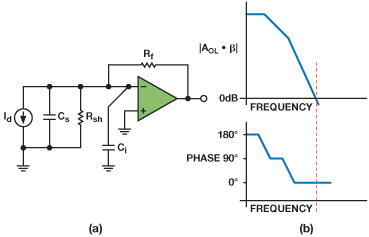Figure 4. Photodiode amplifier model (a) and open loop response (b).

### Selecting External Components for Guaranteed Stability

Figure 4a is a good model for the photodiode amplifier. The system’s open-loop transfer function has one pole at 28 Hz due to the op amp’s open-loop response (from the data sheet) and another pole due to the feedback resistor and the photodiode’s parasitic resistance and capacitance. For our selected component values, this pole occurs at 1 kHz, as shown in Equation 1.(1)

Note that Rsh is two orders of magnitude larger than Rf, so Equation 1 simplifies to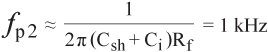(1a)

Each pole causes a 90° phase shift in the open-loop transfer function, for a 180° total phase shift well below the frequency where the open-loop amplitude response crosses 0 dB. As shown in Figure 4b, the lack of phase margin makes it almost certain that the circuit will oscillate.

To ensure stable operation, we can add a zero to the transfer function by placing a capacitor in parallel with Rf. This zero reduces the slope of the transfer function from 40-dB/decade to 20-dB/decade when crossing 0 dB, and results in positive phase margin. The design should have at least 45° of phase margin to guarantee stability. Higher phase margins trade response time for less ringing. The zero added by the capacitor to the open-loop response becomes a pole in the closed-loop response, so the amplifier’s closed-loop response will decrease as the capacitor is increased. Equation 2 shows how to calculate the feedback capacitor for a 45° phase margin.(2)

where fu is the op amp’s unity-gain frequency.

This value for Cf determines the highest practical bandwidth at which the system can operate. Although it’s possible to select a smaller capacitor for lower phase margin and higher bandwidth, the output could ring excessively. Also, all components have tolerances that must be accounted for to guarantee stability under worst case conditions. For our example, we select Cf = 4.7 pF, resulting in a closed-loop bandwidth of 34 kHz, as is typical of many spectroscopy systems.

Figure 5 shows the open-loop frequency response after adding the feedback capacitor. The phase response dips below 30°, but this occurs several decades away from where the gain goes to 0 dB, so the amplifier will remain stable.

### Programmable-Gain TIA

One way to make a photodiode amplifier with programmable gain is to use a transimpedance amplifier with a gain that keeps the output in the linear region even for the brightest light inputs. A programmable-gain amplifier stage can then boost the TIA’s output under low-light conditions, achieving near unity gain for high-intensity signals, as shown in Figure 6a. A second option is to implement the programmable gain directly in the TIA, eliminating the second stage as shown in Figure 6b.Figure 6. (a) TIA first stage followed by a PGA (b) Programmable-gain TIA

### Calculating TIA Noise

The three main sources of noise in a transimpedance amplifier are the op amp’s input voltage noise, its input current noise, and the feedback resistor’s Johnson noise. All of these noise sources are typically expressed as noise density. To convert to units of volts rms, take the noise power (square of the voltage noise density) and integrate over frequency. An accurate and much simpler method is to multiply the noise density times the square root of the equivalent noise bandwidth (ENBW). We can model the amplifier’s closed-loop bandwidth as a first-order response dominated by the feedback resistor, Rf, and the compensation capacitor, Cf. Using the specifications from the stability example, the resulting closed-loop bandwidth is(3)

To convert the 3-dB bandwidth to ENBW in a single-pole system, multiply by π/2:(4)

Now that we have the ENBW, we can find the rms noise due to the feedback resistor and the op amp’s current noise. The resistor’s Johnson noise will appear at the output directly, and the op amp’s current noise will appear as an output voltage after going through the feedback resistor.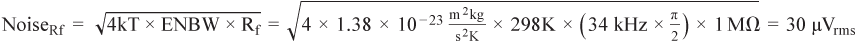(5)(6)

where k is Boltzmann’s constant, and T is the temperature in kelvin.

The final contributor is the op amp’s voltage noise. The output noise is the input noise multiplied by the noise gain. The best way to think about the noise gain for a transimpedance amplifier is to start with the inverting amplifier shown in Figure 7.

For this circuit, the noise gain is(7a)

Using the photodiode amplifier model from Figure 4a, the noise gain will be:(7b)

where Zf is the parallel combination of the feedback resistor and capacitor, and Zin is the parallel combination of the op amp’s input capacitance with the photodiode’s shunt capacitance and shunt resistance.

This transfer function contains several poles and zeroes, and hand analysis would be tedious. However, using the values from the previous examples, we can make some rough approximations. At frequencies near dc, the resistors will dominate, and the gain will be near 0 dB, as the diode’s shunt resistance is two orders of magnitude larger than the feedback resistance. As the frequency increases, the impedance of the capacitors will decrease and start to dominate the gain. Since the total capacitance from the inverting pin of the op amp to ground is much larger than the feedback capacitor, Cf, the gain will start to increase with frequency. Fortunately, the increase in gain does not go on indefinitely, since the pole formed by the feedback capacitor and resistor will stop the increase in gain, and eventually the op amp’s bandwidth will take over to start rolling off the gain.

Figure 8 shows the amplifier’s noise gain behavior over frequency and the location of each pole and zero in the transfer function.

Just as with the resistor noise density, the most accurate way to convert the output noise density of Figure 8 to voltage noise in Vrms is to square the noise density, integrate over the entire frequency spectrum, and then calculate the square root. However, inspection of the response shows that a much easier approach introduces only a small error. For most systems, the first zero and pole occur at relatively low frequencies compared to the second pole. For example, using the specifications from Table 1 and Table 2, the circuit has the following poles and zeroes:(8)(9)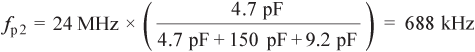(10)

The noise will peak at(11)

Note that fz1 and fp1 occur at a relatively low frequency compared to fp2. Simply assuming that the output noise is equal to the plateau noise (N2 from Equation 11) all the way from dc to fp2 greatly simplifies the math required to calculate the output noise.

With this assumption in mind, the output noise is equal to the input noise density multiplied by the plateau gain and by the ENBW, which is fp2 × π/2:(12)

Now that we have the output referred noise from all three sources, we can combine them to get the overall system output noise. The three noise sources are independent and Gaussian, so we can root-sum-square (RSS) them rather than adding them. When combining terms using RSS, one term will dominate the result if it is more than about three times larger than the others.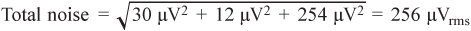(13)

The response of Figure 8 makes it obvious that the noise bandwidth of the op amp is much larger than its signal bandwidth. The additional bandwidth does nothing but contribute to the noise, so we can add a low-pass filter on the output to attenuate the noise at frequencies outside the signal bandwidth. Adding a single-pole RC filter with 34-kHz bandwidth reduces the voltage noise from 254 μVrms to 45 μVrms, and the total noise from 256 μVrms to only 52 μVrms.

### Noise Contributed by Programmable-Gain Stage

If we add a PGA after the transimpedance amplifier, the noise at the output will be the PGA’s noise, plus the TIA’s noise times the additional gain. For example, if we assume an application that requires gains of 1 and 10, and use a PGA with a total input noise density of 10 nV/√Hz, then the output noise due to the PGA will be either 10 nV/√Hz or 100 nV/√Hz.

To calculate the total noise of the system, we can again root-sum-square the TIA’s noise contribution and the PGA’s noise contribution as shown in Table 3. For this example, assume that the PGA includes a 34-kHz filter. As we can see, for a gain of 10, the TIA’s noise contribution will appear at the PGA’s output multiplied by the PGA gain.

Table 3. Total System Noise for TIA + PGA Architecture

 Noise at PGA Input Output Noise G = 1 Output Noise G = 10 TIA with RC Filter 52 μVrms 52 μVrms 520 μVrms PGA with 34-kHz BW 2.3 μVrms 2.3 μVrms 23.1 μVrms RSS Noise Total 52 μVrms 524 μVrms

As we would expect, the output noise is slightly more than ten times as large when operating with a gain of ten than when the PGA is set for a gain of one.

### Noise Advantage of a Single Gain Stage

An alternative approach is to use a transimpedance amplifier with programmable gain, skipping the PGA stage altogether. Figure 9 shows a theoretical circuit with two programmable transimpedance gains of 1 MΩ and 10 MΩ. Each transimpedance resistor needs its own capacitor to compensate for the photodiode’s input capacitance. To be consistent with the previous example, we will keep the signal bandwidth at 34 kHz for both gain settings. This means selecting a 0.47-pF capacitor in parallel with the 10-MΩ resistor. In this case, the output voltage noise, when operating with the 1-MΩ resistor, is the same as in Equation 12. When operating with a transimpedance gain of 10 MΩ, the larger resistor results in higher Johnson noise, higher current noise (the current noise is now multiplied by 10 MΩ instead of 1 MΩ), and higher noise gain. Following the same methodology, the three main noise contributors are(14)(15)(16)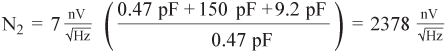(17)(18)

and the total output noise is(19)

Adding a single-pole RC filter with a bandwidth of 34 kHz at the output results in lower noise, for a total system noise of 460 μVrms. Due to the higher gain, fp2 is much closer to the signal bandwidth, so the noise reduction is not as dramatic as when operating with a gain of 1 MΩ.

Table 4 shows a summary of the noise performance for both amplifier architectures. For a transimpedance gain of 10 MΩ, the total noise will be about 12% lower than with the two-stage circuit.

Table 4. Total System Noise Comparison

 Output Noise, Programmable TIA Output Noise, TIA Followed by PGA Gain = 1 52 μVrms 52 μVrms Gain = 10 460 μVrms 524 μVrms

### Programmable-Gain Transimpedance Amplifier

Figure 9 shows a programmable-gain transimpedance amplifier. It’s a good concept, but the on resistance and leakage current of the analog switches will introduce errors. The on resistance causes voltage and temperature dependent gain errors and the leakage current causes offset errors, especially at high temperatures.

The circuit in Figure 10 avoids these problems by using two switches in every transimpedance leg. Although this requires twice as many switches, the on resistance of the switches on the left is within the feedback loop, so the output voltage depends only on the current through the selected resistor. The switches on the right look like an output impedance and will contribute negligible error if the amplifier drives a high-impedance load, such as an ADC driver.

The circuit of Figure 10 will work for dc and low frequencies, but the parasitic capacitance across the switches in the off state presents yet another challenge. These parasitic capacitors, labeled Cp in Figure 10, connect the unused feedback path to the output, so they will decrease the overall bandwidth. Figure 11 shows how these capacitors end up connecting to the unselected gain leg, effectively changing the transimpedance gain to be a combination of the selected gain in parallel with an attenuated version of the unselected gain.

Depending on the desired bandwidth and feedback resistor, the parasitic capacitance could result in a significant difference between the expected and measured behavior of the amplifier. For example, assume the amplifier of Figure 11 uses the same 1 MΩ and 10 MΩ values as in our previous circuit, with their respective capacitors of 4.7 pF and 0.47 pF, and we select the 10 MΩ gain. If each switch has approximately 0.5 pF of feed-through capacitance, Figure 12 shows the difference between the ideal and actual bandwidths, taking into account the parasitic path.

One way to address this problem is to replace each switch with two switches in series. This reduces the parasitic capacitance by half at the expense of additional components. Figure 13 shows this approach.

If the application requires even more bandwidth, a third alternative is to use SPDT switches to connect every unused input to ground. Although the parasitic capacitance from each open switch is still in the circuit, Figure 14b shows how each parasitic capacitor appears to be connected from the output of the op amp to ground or from the end of the unused feedback leg to ground. Capacitance from the amplifier output to ground tends to be associated with instability and ringing, but in this case, the total parasitic capacitance of just a few picofarads will not have a significant effect on the output. The parasitic capacitance that appears from the inverting input to ground will add to the photodiode’s shunt capacitance and the op amp’s own input capacitance and will represent a negligible increase when compared with the photodiode’s large shunt capacitance. Assuming 0.5 pF of feed-through capacitance for each switch, the op amp would see an additional 2-pF load on its output, which most op amps will drive with no problems.